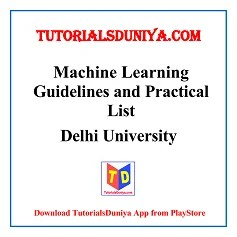## Machine Learning Guidelines and Practical List

Course Objective: The course aims at introducing the basic concepts and techniques of machine learning so that a student can apply machine learning techniques to a problem at hand.

### Machine Learning Guidelines

Introduction: Basic definitions, Hypothesis space and inductive bias, Bayes optimal classifier and Bayes error, Occam’s razor, Curse of dimensionality, dimensionality reduction, feature scaling, feature selection methods.

Regression: Linear regression with one variable, linear regression with multiple variables, gradient descent, logistic regression, over-fitting, regularization. performance evaluation metrics, validation methods.

Classification: Decision trees, Naive Bayes classifier, k-nearest neighbor classifier, perceptron, multilayer perceptron, neural networks, back-propagation algorithm, Support Vector Machine (SVM), Kernel functions.

Clustering: Approaches for clustering, distance metrics, K-means clustering, expectation maximization, hierarchical clustering, performance evaluation metrics, validation methods.

Machine Learning Reference Books:

1. Flach, P. (2015). Machine Learning: The Art and Science of Algorithms that Make Sense of  Data. Cambridge University Press.

2. Mitchell, T.M. (2017). Machine Learning. McGraw Hill Education.

### Machine Learning Practicals List

1. Perform elementary mathematical operations in Octave/MATLAB like addition, multiplication, division and exponentiation.

2. Perform elementary logical operations in Octave/MATLAB (like OR, AND, Checking for Equality, NOT, XOR).

3. Create, initialize and display simple variables and simple strings and use simple formatting for variable.

4. Create/Define single dimension / multi-dimension arrays, and arrays with specific values like array of all ones, all zeros, array with random values within a range, or a diagonal matrix.

5. Use command to compute the size of a matrix, size/length of a particular row/column, load data from a text file, store matrix data to a text file, finding out variables and their features in the current scope.

6. Perform basic operations on matrices (like addition, subtraction, multiplication) and display specific rows or columns of the matrix.

7. Perform other matrix operations like converting matrix data to absolute values, taking the negative of matrix values, additing/removing rows/columns from a matrix, finding the maximum or minimum values in a matrix or in a row/column, and finding the sum of some/all elements in a matrix.

8. Create various type of plots/charts like histograms, plot based on sine/cosine function based on data from a matrix. Further label different axes in a plot and data in a plot.

9. Generate different subplots from a given plot and color plot data.

10. Use conditional statements and different type of loops based on simple example/s.

11. Perform vectorized implementation of simple matrix operation like finding the transpose of a matrix, adding, subtracting or multiplying two matrices.

12. Implement Linear Regression problem. For example, based on a dataset comprising of existing set of prices and area/size of the houses, predict the estimated price of a given house.

13. Based on multiple features/variables perform Linear Regression. For example, based on a number of additional features like number of bedrooms, servant room, number of balconies, number of houses of years a house has been built – predict the price of a house.

14. Implement a classification/ logistic regression problem. For example based on different features of students data, classify, whether a student is suitable for a particular activity. Based on the available dataset, a student can also implement another classification problem like checking whether an email is spam or not.

15. Use some function for regularization of dataset based on problem 14.

16. Use some function for neural networks, like Stochastic Gradient Descent or backpropagation – algorithm to predict the value of a variable based on the dataset of problem 14.

Source: https://www.du.ac.in